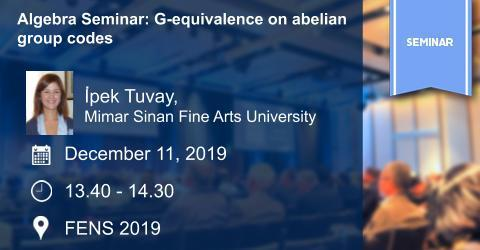## Algebra Seminar: G-equivalence on abelian group codes

### Algebra Seminar: G-equivalence on abelian group codesSpeaker: İpek Tuvay, Mimar Sinan Fine Arts University

Title: G-equivalence on abelian group codes

Date/Time: December 11, 2019  /  13.40-14.30

Place: FENS 2019

Abstract: Let G be a finite abelian group and F a field with characteristic coprime to the order of G. A G-code over F is defined as an ideal of the group algebra FG. In this talk, first the notion of G-equivalence on the set of G-codes will be introduced, then the basic properties of this notion will be discussed. Ferraz, Guerreiro and Milies introduce a correspondence between the set of minimal G-codes and the set of some special subgroups of G. By proving that the notion of G-isomorphism coincides with the notion of isomorphism on these subgroups together with the correspondence mentioned above, we calculate the number of G-equivalence classes of minimal G-codes for some specific families of abelian groups G. We also prove that the number of G-equivalence classes of minimal G-codes is equal to the number of divisors of the exponent of G if and only if for each prime p dividing the order of G the Sylow p-subgroups of G are homocyclic. This is a joint work with Fatma Altunbulak Aksu.

Contact: Michel Lavrauw